Smartick is a fun way to learn math!Apr21

Subtraction With and Without Carrying

Today we are going to learn how to solve subtraction problems both with and without carrying. We will begin with a review of what subtraction is and its elements, and then we will see an example of each type of subtraction with a video.

Index

What is Subtraction?

Subtraction is a mathematical operation that consists of taking, removing, or reducing something from a whole. Subtracting is one of the basic mathematical operations along with addition, which is its inverse process.

To put it simply we can say that when we are subtracting we are removing a quantity from a set of objects.

If you would like to review subtraction and practice with some examples, take a look at our post about subtraction.

Elements of Subtraction

Minuend

This is the amount that we are starting with, the number that another number (subtrahend) is being subtracted from for us to get the difference.

Subtrahend

This is the amount to subtract or remove, from the minuend to get the difference.

How to Teach Subtraction to a Primary School Student

In Smartick we use diverse digital manipulative materials in order to demonstrate mathematical concepts to children that are often very abstract.

This time we are going to use blocks that can be used alone (as units), grouped into bars of ten (tens), or grouped into larger quantities (hundreds, thousands). If you don’t have these blocks at home you can use things like popsicle sticks or toothpicks and create groups of ten or more using rubber bands to hold them together.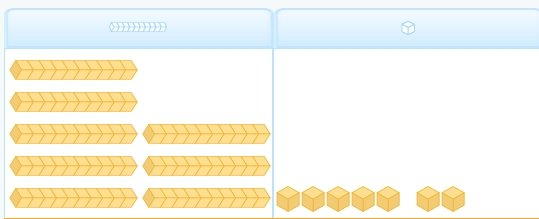In this case, we have represented the number that has 8 bars of ten and 7 units, which equals 87.

Subtraction without Carrying

To teach subtraction without carrying we follow a few steps. If you follow them, learning to subtract will be very easy.

We are going to explain the steps for vertical subtraction. This is not the only way to subtract, but it is the most common. Of course, subtraction can be done many other ways – mentally, horizontally – but today we are going to look at it vertically.

Steps to Solve Subtraction Without Carrying

1. Place the subtrahend (the number that will be subtracted) underneath the minuend (the number that will be subtracted from) so that the units and tens are in the same column.
2. Place the material needed to represent the minuend, separated into columns. Leave space for the subtrahend.
3. Take away the material in the units column indicated by the subtrahend, representing the subtraction.
4. Take away the material in the tens column, also representing the operation.

To get a better understanding, let’s look at it with blocks.

Example of Subtraction Without Carrying

We are going to subtract 67 from 79, in other words, 79 – 67.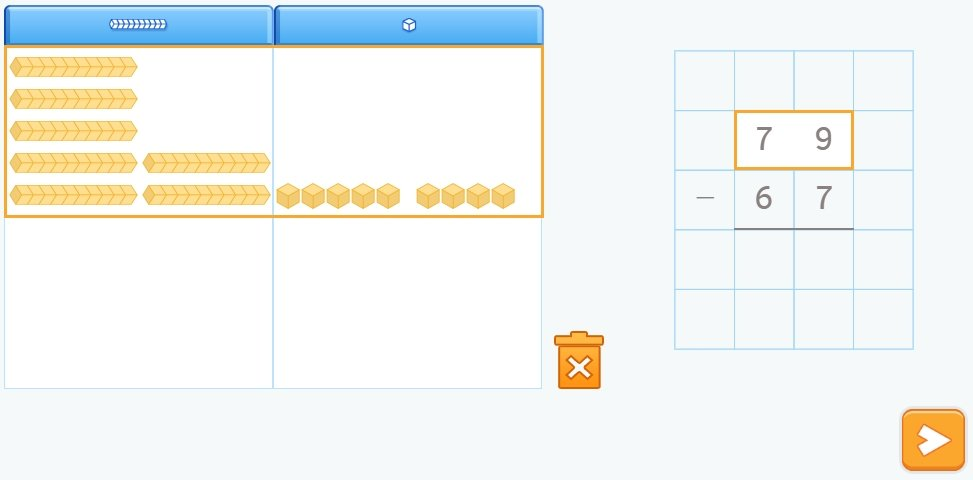With the manipulative material, we have represented the minuend, 79, with 7 bars (tens) and 9 loose cubes (units).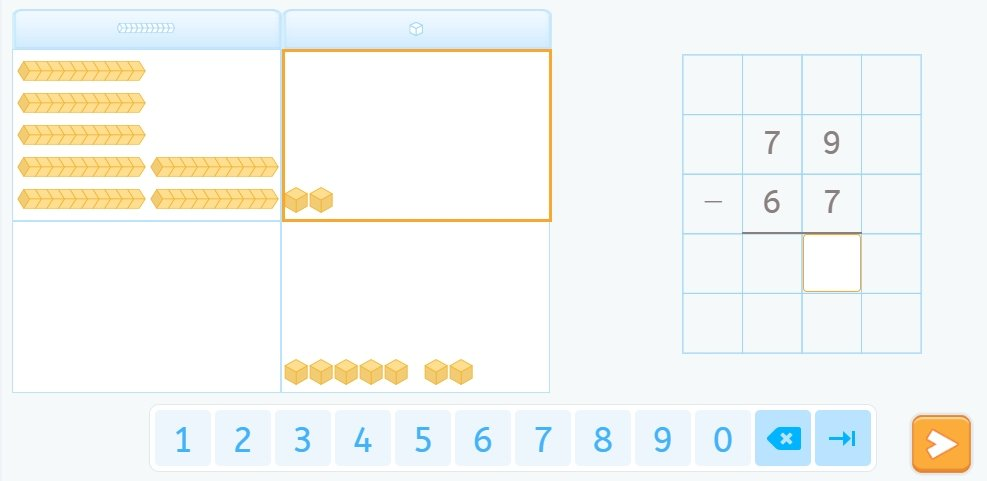We move 7 units to the block below, that is we ”take away” the 7 indicated by the subtrahend from the minuend.

How many units are left? 2, we write the result beneath the units.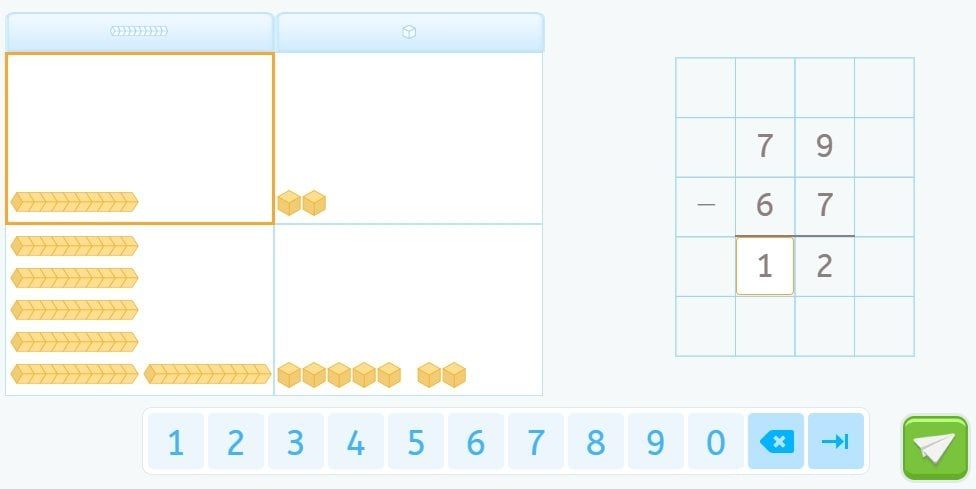We remove 6 tens or bars from the minuend and have one left.

We write the one in the result and we’ve finished.

Video to Learn About Subtraction Without Carrying

To better understand subtraction without carrying, take a look at the following video of Leo and his friends going to the bowling alley.

This is a video of one of our interactive tutorials. Although it is not interactive this way, you can still watch it as many times as you need to and share it with friends. If you would like to access our interactive tutorials, register with Smartick! The online method that helps children ages 4 to 14 learn and practice mathematics.

Subtraction With Carrying

What we have seen so far is great, but it does not always work.

Why? Because the subtraction that we are doing is with natural numbers and the results need to be positive. But it may happen that some of the numbers being subtracted are larger than the minuend. We are talking about subtraction with carrying.

You will see that with a little care and support from examples – both manipulative and visual that we have in Smartick – you’ll have no problem solving these exercises. Here is how to subtract with carrying.

How to Solve Subtraction With Carrying

Since we have been seeing double-digit numbers, the number of units in the subtrahend needs to be larger than the units in the minuend.

In numbers with more digits, you may have to carry in other positions too.

But we are going to start with an example where we use carrying in the tens.

We are going to solve 52 – 17.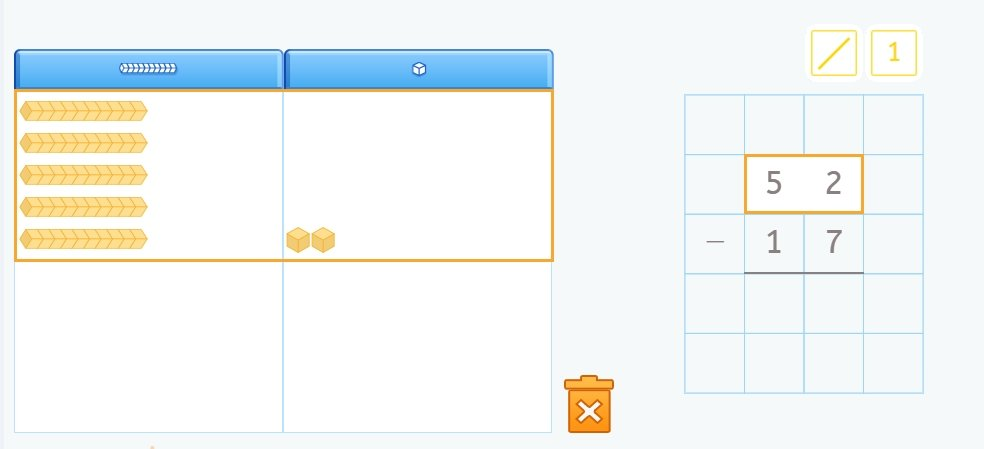Until now, everything seems the same as subtracting without carrying.

The only problem is that if we would like to do the same as before, we will need to take away 7 units from a position where there are only 2.

How do we do it?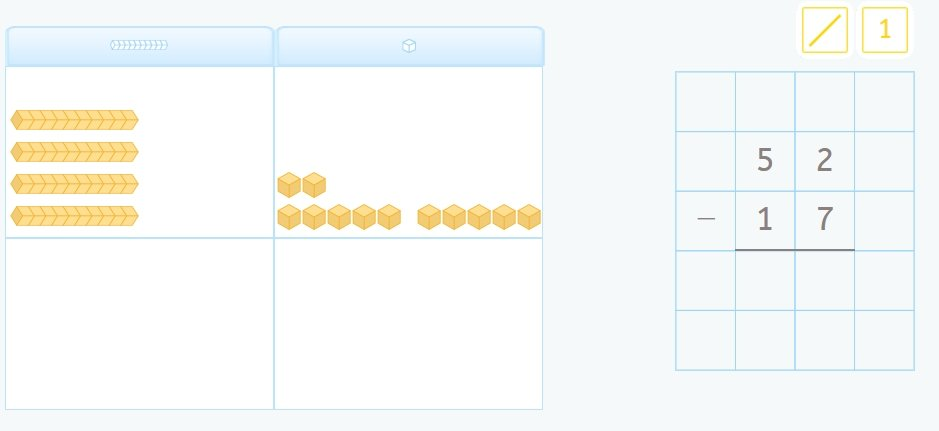The blocks of tens can be broken down into 10 loose blocks!

This is what we do to change a group of ten into ten units.

Now we have 4 tens and 12 units.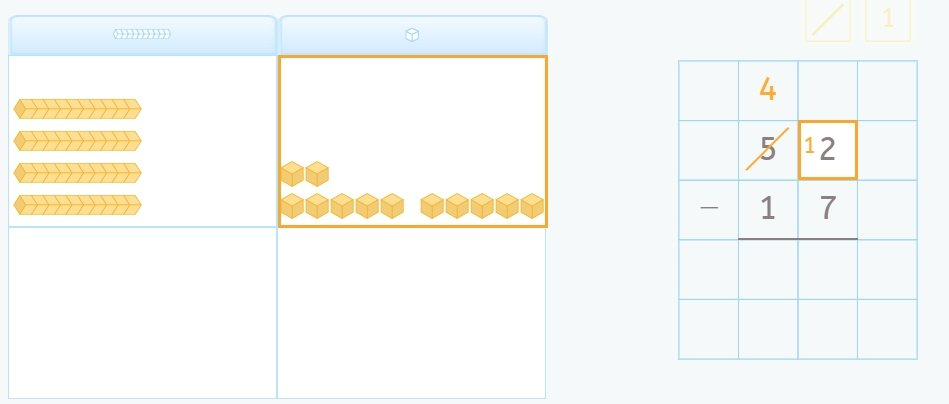Now we write our result that we arrived at with the manipulatives.

Since we don’t have 5 tens, we have 4, we cross out the 5 and write 4.

As for the units, we no longer have 2 units and have added 10 more, so we write 1 to represent that we now have 12 units.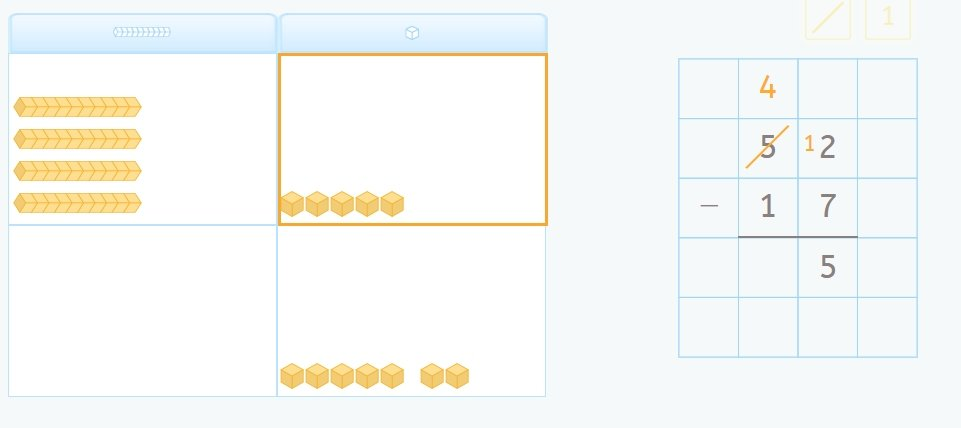We continue as we did with the subtraction without carrying since we have enough units now.

We bring down the 7 and write in the result that we have 5 left.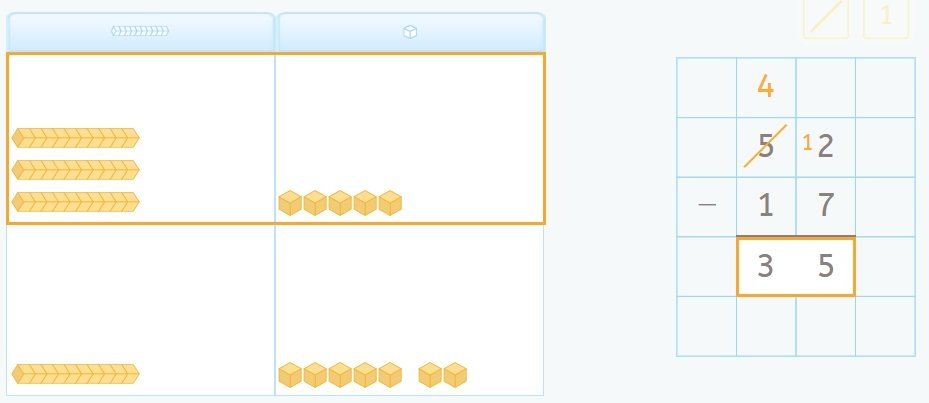We do the same with the tens, taking away 1, leaving us with 3.

We write it down in the operation and…

…we’ve finished!

Video to Learn about Subtraction with Carrying

The exercises in this post were taken from Smartick’s Subtraction Tutorial. Our tutorials are interactive but we have included some in this blog post as videos so you can still watch them as many times as you need to and share them with friends. If you would like to access our interactive tutorials, register with Smartick!

Fun is our brain’s favorite way of learning
Diane Ackerman
Smartick is a fun way to learn math
• 15 fun minutes a day
• Millions of students since 2009